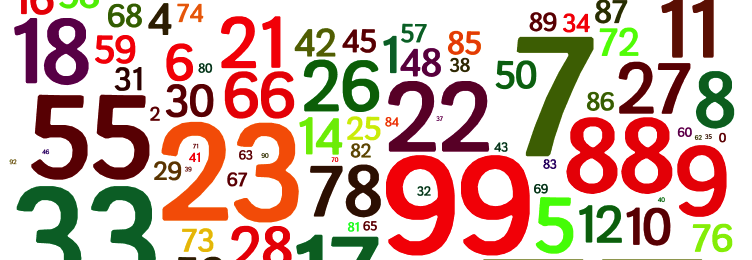# How to Generate Random Number in C and C++?

Here you will get program and learn how to generate random number in C and C++.

We can use rand() function to generate random number. It is defined in stdlib.h header file. It generates a random number between 0 and RAND_MAX (both inclusive). Here RAND_MAX is a constant whose value depends upon the compiler you are using.

The random value returned by rand() depends upon the initial value called as seed. The value of seed is same each time you run the program. Due to this the sequence of random numbers generated in each program run are same.

To solve this problem we provide new seed value on each program run. You can give seed value to rand() function algorithm using srand() function. We give current time in seconds as an argument of srand() to give a unique seed value for each program run.

Note: Using rand() is not a secure way of generating random number if you are developing a real world application. To generate a secure random number read this.## Program to Generate Random Number in C and C++

C Program

C++ Program

### How to generate random number in a range?

Suppose there are two numbers a and b. Then result of a%b will be always in between 0 and b-1 (both inclusive). So we will use this concept to generate random number in a range.

Lets say you want to generate number in between 1 and 10 then it can be done by rand()%10+1.

Comment below if you have doubts or found anything incorrect in above tutorial for random number in C and C++.

### 4 thoughts on “How to Generate Random Number in C and C++?”

1.Hello sir I did not understand the meaning of line
Srand(time(null));
1.2.3.